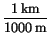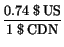Next: Questions Up: Introduction Previous: Symmetry

# Units and measurements

One of the crucial aspects of science is the measurement process, which provides the ultimate means to accept or reject a theory. Quantitative measurements are also important in other areas - for example, given that we are being constantly exposed to radiation all around us, what makes an X-ray particularly dangerous? For this we have to discuss how science measures quantities.

There are five basic units that we shall be concerned with. These are, together with how they are measures in the metric (SI) system, are summarized in the table below.

Table 1.3: Basic units
 Quantity Unit Abbreviation Length meter m Time second s Mass kilogram kg Temperature o Celsius o C Charge Coulomb C

Probably the first four are familiar to us; the last one, the unit of charge, we shall encounter when we discuss electricity.

It is a good idea to have an intuitive idea of the relative sizes of these units - a person might be 2 m tall, have a mass of 70 kg, etc. However, for some quantities, such as the age of a person, or how far it is to Paris, these fundamental units may be inconvenient due to the large or small numbers involved. For this reason we often will use prefixes attached to these basic units to denote some multiple of them. Some of the more common prefixes are given in the table below.

Table 1.4: Common prefixes
 Prefix Factor of 10 nano 10-9 milli 10-3 centi 10-2 kilo 103 mega 106

Here we have also used scientific notation, whereby, for example, 103 = 10 x 10 x 10 = 1000, and 10-3 = 1 / ( 10 x 10 x 10 ) = 0.001. Thus, for example, 1 km = 1000 m. To convert units, one simply multiplies by the relevant conversion factor, as, for example,

 3000 m = 3000 m x= 3 km.

This also works at converting into other systems of units; for example, using 2.54 cm = 1 in, we can find
 12 in = 12 in x= 30.48 cm

or even, using 1 $CDN = 0.74$ US,
 20  $CDN = 20$ CDN x= 14.8  \$ USNext: Questions Up: Introduction Previous: Symmetry
modtech@theory.uwinnipeg.ca
1999-09-29